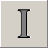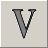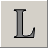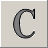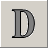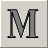﻿﻿ Roman numerals - Collection Studio

Collection Studio 4.75

[ Data: ﻿31 sierpnia 2018 ]

Panstwa opinia

Usability of the CollectionStudio is:

Zobaczyć wynik

Roman numerals

Collection Studio » Dates Calculator » Roman numerals

The Romans were active in trade and commerce, and from the time of learning to write they needed a way to indicate numbers. The system they developed lasted many centuries, and still sees some specialized use today.

Roman numerals traditionally indicate the order of rulers or ships who share the same name (i.e. Queen Elizabeth II). They are also sometimes still used in the publishing industry for copyright dates, and on cornerstones and gravestones when the owner of a building or the family of the deceased wishes to create an impression of classical dignity. The Roman numbering system also lives on in our languages, which still use Latin word roots to express numerical ideas. A few examples: unilateral, duo, quadricep, septuagenarian, decade, milliliter.

The big differences between Roman and Arabic numerals (the ones we use today) are that Romans didn't have a symbol for zero, and that numeral placement within a number can sometimes indicate subtraction rather than addition.

The Simple Principle

Roman numerals are mathematically converted to Arabic numerals simply through the assignment of an Arabic numerical value to each letter and calculating a total: M=1000 | D=500 | C=100 | L=50 | X=10 | V=5 | I=1.

Here are the basics:

I

The easiest way to note down a number is to make that many marks - little I's. Thus I means 1, II means 2, III means 3. However, four strokes seemed like too many....

20th Century

1901 = MCMI
1902 = MCMII
1903 = MCMIII
1904 = MCMIV
1905 = MCMV
1906 = MCMVI
1907 = MCMVII
1908 = MCMVIII
1909 = MCMIX
1910 = MCMX

1911 = MCMXI
1912 = MCMXII
1913 = MCMXIII
1914 = MCMXIV
1915 = MCMXV
1916 = MCMXVI
1917 = MCMXVII
1918 = MCMXVIII
1919 = MCMXIX
1920 = MCMXX

1921 = MCMXXI
1922 = MCMXXII
1923 = MCMXXIII
1924 = MCMXXIV
1925 = MCMXXV
1926 = MCMXXVI
1927 = MCMXXVII
1928 = MCMXXVIII
1929 = MCMXXIX
1930 = MCMXXX

1931 = MCMXXXI
1932 = MCMXXXII
1933 = MCMXXXIII
1934 = MCMXXXIV
1935 = MCMXXXV
1936 = MCMXXXVI
1937 = MCMXXXVII
1938 = MCMXXXVIII
1939 = MCMXXXIX
1940 = MCMXL

1941 = MCMXLI
1942 = MCMXLII
1943 = MCMXLIII
1944 = MCMXLIV
1945 = MCMXLV
1946 = MCMXLVI
1947 = MCMXLVII
1948 = MCMXLVIII
1949 = MCMXLIX
1950 = MCML

1951 = MCMLI
1952 = MCMLII
1953 = MCMLIII
1954 = MCMLIV
1955 = MCMLV
1956 = MCMLVI
1957 = MCMLVII
1958 = MCMLVIII
1959 = MCMLIX
1960 = MCMLX

1961 = MCMLXI
1962 = MCMLXII
1963 = MCMLXIII
1964 = MCMLXIV
1965 = MCMLXV
1966 = MCMLXVI
1967 = MCMLXVII
1968 = MCMLXVIII
1969 = MCMLXIX
1970 = MCMLXX

1971 = MCMLXXI
1972 = MCMLXXII
1973 = MCMLXXIII
1974 = MCMLXXIV
1975 = MCMLXXV
1976 = MCMLXXVI
1977 = MCMLXXVII
1978 = MCMLXXVIII
1979 = MCMLXXIX
1980 = MCMLXXX

1981 = MCMLXXXI
1982 = MCMLXXXII
1983 = MCMLXXXIII
1984 = MCMLXXXIV
1985 = MCMLXXXV
1986 = MCMLXXXVI
1987 = MCMLXXXVII
1988 = MCMLXXXVIII
1989 = MCMLXXXIX
1990 = MCMXC

1991 = MCMXCI
1992 = MCMXCII
1993 = MCMXCIII
1994 = MCMXCIV
1995 = MCMXCV
1996 = MCMXCVI
1997 = MCMXCVII
1998 = MCMXCVIII
1999 = MCMXCIX
2000 = MM

2001 = MMI
2002 = MMII
2003 = MMIII
2004 = MMIV
2005 = MMV
2006 = MMVI
2007 = MMVII
2008 = MMVIII
2009 = MMIX
2010 = MMX

2011 = MMXI
2012 = MMXII
2013 = MMXIII
2014 = MMXIV
2015 = MMXV
2016 = MMXVI
2017 = MMXVII
2018 = MMXVIII
2019 = MMXIX
2020 = MMXX

V

So the Romans moved on to the symbol for 5 - V. Placing I in front of the V - or placing any smaller number in front of any larger number - indicates subtraction. So, IV means 4. After V comes a series of additions - VI means 6, VII means 7, VIII means 8.

X

X means 10. But wait - what about 9? Same deal. IX means to subtract I from X, leaving 9. Numbers in the teens, twenties and thirties follow the same form as the first set, only with X's indicating the number of tens. So XXXI is 31, and XXIV is 24.

L

L means 50. Based on what you've learned, I bet you can figure out what 40 is. If you guessed XL, you're right = 10 subtracted from 50. And thus 60, 70, and 80 are LX, LXX and LXXX.

C

C stands for centum, the Latin word for 100. A centurion led 100 men. We still use this in words like "century" and "cent." The subtraction rule means 90 is written as XC. Like the X's and L's, the C's are tacked on to the beginning of numbers to indicate how many hundreds there are: CCCLXIX is 369.

D

D stands for 500. As you can probably guess by this time, CD means 400. So CDXLVIII is 448.

M

M is 1,000. You see a lot of Ms because Roman numerals are used a lot to indicate dates.

V

Larger numbers were indicated by putting a horizontal line over them, which meant to multiply the number by 1,000. Hence the V at left has a line over the top, which means 5,000. This usage is no longer current, because the largest numbers usually expressed in the Roman system are dates, as discussed above.

Online Roman numerals converter

Press roman numbers shown on your coin:

Roman year conversion